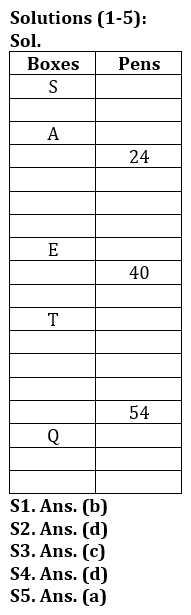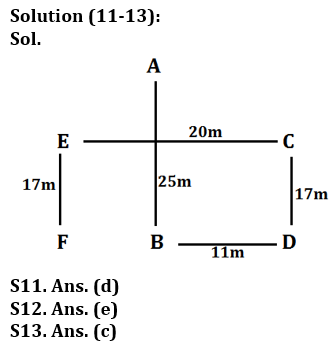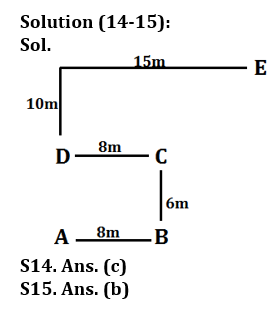Latest Banking jobs   »

# Reasoning Ability Quiz For LIC AAO Mains 2023-12th March

Direction (1-5): Study the following information carefully and answer the questions given below:
A Certain number of boxes are placed one above another. Each box has different number of Pens. Four boxes are placed between A and E. Not more than three boxes are placed above A. Three boxes are placed between E and the box which has 24 pens which is placed above E. The Box S is placed at topmost position. The number of boxes placed between box A and the box which has 40 pens is half of the number of boxes placed below box E. There are four boxes placed between the box which has 24 pens and the box which has 16 more pens than that box. The number of boxes are placed between E and T is one less than the number of boxes between T and the box which has 54 pens. Box Q is placed 3rd from the bottom most position. The box which has 54 pens is placed just above Q. The number of boxes placed between T and Q is the four times than the number of boxes placed between S and A.

Q1. How many boxes are placed between S and T?
(a) Eight
(b) Nine
(c) Six
(d) Five
(e) None

Q2. If box M is placed between T and the box which has 40 pens, then find the position of the box M from the topmost position?
(a) 6th
(b) 8th
(c) 12th
(d) 10th
(e) 4th

Q3. What is the total number of boxes in the arrangement?
(a) Eighty
(b) Nineteen
(c) Eighteen
(d) Twenty
(e) Can’t be determined

Q4. The number of boxes placed between S and E is same as the number of boxes placed between Q and the box ___?
(a) S
(b) The box which has 24 pens
(c) A
(d) The box which has 40 pens
(e) Both (a) and (d)

Q5. What is the product of the number of pens of boxes which is placed 4th from the topmost position and 4th from the bottommost position?
(a) 1296
(b) 1225
(c) 3025
(d) 788
(e) None of these

Directions (6-10): Answer the questions based on the information given below.
In a certain language,
‘Control beyond world seem’ is coded as ‘R5, H5, H3, R6’
‘During lock period maintain’ is coded as ‘R4, H4, X5, D5’
‘Shall the report execute’ is coded as ‘K3, H5, A4, K5’
‘Without failure done work’ is coded as ‘L5, R3, D4, R4’

Q6. What is the code for ‘JUNIOR’?
(a) N11
(b) R3
(c) Y7
(d) X4
(e) J1

Q7. What is the code for ‘REVIEWING’?
(a) R5
(b) H6
(c) U9
(d) X2
(e) D8

Q8. Which of the following word is coded as ‘X3’?
(a) CUBE
(b) METER
(c) FORD
(d) BRAIN
(e) WASH

Q9. What is the code for ‘COUNT’?
(a) S4
(b) P7
(c) O2
(d) T5
(e) R4

Q10. Which of the following word is coded as ‘H4’?
(a) CUBE
(b) METER
(c) SHALL
(d) STEEL
(e) PAPER

Directions (11-13): Study the following information carefully and answer the questions given below.
P%Q (10)- P is 12m in north of Q
P\$Q (15)- P is 17m in south of Q
P#Q (22)- P is 24m in east of Q
P&Q (14)- P is 16m in west of Q
A%B (23), D#B (9), C%D (15), E&C (18), F\$E (15)

Q11. C is in which direction with respect to B?
(a) North
(b) West
(c) North-west
(d) North-East
(e) South-west

Q12. What is the distance between F and D?
(a) 12m
(b) 14m
(c) 16m
(d) 17m
(e) 20m

Q13. What is the direction of E with respect to D?
(a) North
(b) East
(c) North-West
(d) South-East
(e) None of these

Directions (14-15): Study the following information carefully and answer the given questions.
Rihan starts walking from point A in east direction and walks 8m to reach at point B. From point B, he takes a left turn and walks 6m and reach at point C, then again, he turns his left and walks 8m to reach at point D. From point D he takes two consecutive right turns and walks 10m and 15m respectively to reach at point E.

Q14. What is the shortest distance between point A and point C?
(a) 12m
(b) 16m
(c) 10m
(d) 15m
(e) None of these

Q15. What is the direction of point E with respect to point D?
(a) South-west
(b) North-east
(c) East
(d) North-west
(e) None of these

SolutionsSolution (6-8):
Sol. The coding language used to code the given words should be decoded in two steps,
Step I: The alphabet is third succeeding alphabet of second alphabet (from left) of the word.
Step II: The number is one plus the number of consonants in the word.
For example: CONTROL is coded as ‘R6’
Alphabet: O + 3 = R
Number: Number of consonants + 1 = 5 + 1 = 6
SO, the code for ‘CUBE’ is X3.
The code for ‘METER’ is ‘H4’
The code for ‘FORD’ is ‘R4’
The code for ‘BRAIN’ is ‘U4’.
The code for ‘WASH’ is ‘D4’ and so on…

S6. Ans. (d)
S7. Ans. (b)
S8. Ans. (a)
S9. Ans. (e)
S10. Ans. (b)## FAQs

### When will the LIC AAO Mains exam be held?

LIC AAO Mains exam will be held on 18 March

#### Congratulations!Union Budget 2023-24: Free PDF